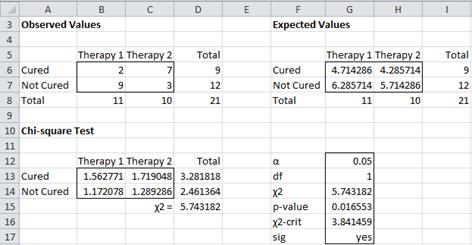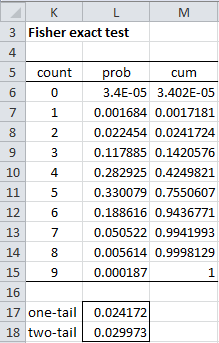Hits: 658

Fisher’s Exact Test

When the conditions for Pearson’s chi-square test are not met, especially when one of more of the cells have expi < 5, an alternative approach with 2 × 2 contingency tables is to use Fisher’s exact test. Since this method is more computationally intense, it is best used for smaller samples.

Example 1: Repeat Example 2 from Independence Testing using the data in range A5:D8 of Figure 1; i.e. determine whether the cure rate is independent of the therapy used.Figure 1 – Data and Chi-square test for Example 1

As you can see from Figure 1, the expectation for two of the cells is less than 5. Since we are dealing with a 2 × 2 contingency table with relatively small sample size, it is better to use Fisher’s exact test.

The approach is to determine how many different ways the above marginal frequencies can be achieved and then determine the probability that the above observed cell configuration can be obtained merely by chance.

We can restrict our attention to any one of the cells since once the frequency for one cell is determined the frequencies for the other cells can be determined from the marginal totals. We choose cell B6 since it has the smallest marginal total (namely 9 in cell D6) and it is smaller than the other element that makes up this marginal total (namely 7 in cell C6).

Now cell B6 can take any value between 0 and 9; once this value is set the values of the other three cells can be adjusted to maintain the marginal totals.

The probability that cell B6 takes on a specific value x is equivalent to the probability of getting x successes in a sample of size 9 (cell D6) taken without replacement from a population of size 21 (cell D8) which contains 11 (cell B8) successful choices. This can be calculated by the hypergeometric distribution. Here cells D6 and B8 are cells with the marginal totals corresponding to cell B6 and cell D8 contains the grand total.

Figure 2 contains a table of the probabilities for each possible value of x.Figure 2 – Fisher exact test for Example 1

Thus, e.g., cell L11 contains the formula

=HYPGEOMDIST(K11,\$B\$8,\$D\$6,\$D\$8)

Our test consists of determining whether the probability that at most 2 of those taking therapy 1 are cured (the observed count in cell B6) is less than .05. From Figure 2, we see that the probability of count 0 is 3.4E-05, the probability of count 1 is .001684 and the probability of count 2 is .022454 for a cumulative probability of .024172 < .05 = α, and so we reject the null hypothesis and conclude there is a significant difference between the cure rates for the two therapies.

There are one-tail and two-tail versions of the test. The p-value for the one tail test (cell L17) is given by the formula =SUM(L6:L8) or equivalently (for versions of Excel starting with Excel 2010)

= HYPGEOM.DIST(K8,B8,D6,D8,TRUE)

The p-value for the two tail test (cell L18) given by the formula

=SUM(L6:L8)+SUM(L14:L15)

where K14 is the leftmost cell in the right tail that has a pdf value ≤ L8 (since .005614 ≤ .022454, but .050522 > .022454). Equivalently, we can use the formula (for versions of Excel starting with Excel 2010)

= HYPGEOM.DIST(K8,B8,D6,D8,TRUE)+1−HYPGEOM.DIST(K13,B8,D6,D8,TRUE)

Real Statistics Excel Function: The following function is provided in the Real Statistics Resource Pack:

FISHERTEST(R1, tails) = the probability calculated by the Fisher Exact Test for a 2 × 2,  2 × 3, 2 × 4, 2 × 5, 2 × 6, 2 × 7, 2 × 8, 2 × 9, 3 × 3, 3 × 4 or 3 × 5 contingency table contained in range R1.

The range R1 must contain only numeric values. For a 2 × 2 contingency table, there is an optional second argument, tails = 1 (one-tailed test) or 2 (two tailed test, default). For other sized contingency tables only the p-value of the two-tailed test can be returned.

For Example 1, FISHERTEST(B6:C7,1) = .024172 and FISHERTEST(B6:C7, 2) = .029973.

Observation: Because the Fisher Exact tests can be resource intensive, limits have been placed on the sum of all the cells in the supported contingency tables. These limits are currently set at 2,000 for a 2 × 3 table, 1,250 for a 2 × 4 table, 360 for a 2 × 5 table, 175 for a 2 × 6 table, 110 for a 2 × 7 table, 75 for a 2 × 8 table, 40 for a 2 × 9 table, 320 for a 3 × 3 table, 95 for a 3 × 4 table and 30 for a 3 × 5 table. There are no limits for 2 × 2 tables.

If you want to exceed these limits, you can add a third argument to the FISHERTEST function which describes how much you want to increase the limit. E.g. if you want to use the Fisher exact test for a 3 × 3 contingency table in range A1:C3 the sum of whose cells is 350, then you can use the array formula =FISHERTEST(A1:C3,,1.1). The 1.1 specifies that you have increased the limit for a 3 × 3 contingency table from 320 to 320 × 1.1 = 352. Since 350 < 352, the function will run, although it will take a little longer. Similarly, you can use =FISHERTEST(A1:C3,,1.5) for any 3 × 3 contingency table whose entries sum to at most 320 × 1.5 = 480. When the sum is 480, expect the processing to take some time.

Example 2: Determine whether being pro-choice or pro-life is independent of a US citizen’s political party based on the sample shown in range A3:D7 of Figure 3.

We can use the Fisher Exact Test by using the formula =FISHERTEST(B4:C6). The result, as shown in cell H13 of Figure 3, is that being pro-choice or pro-life is not independent of party affiliation since p-value = 4.574E-06 < .05 = α (two-tailed test).How to determine Spearman’s correlation in Python

Statistics for Beginners in Excel – Fisher’s Exact Test

Free Machine Learning & Data Science Coding Tutorials in Python & R for Beginners. Subscribe @ Western Australian Center for Applied Machine Learning & Data Science.

Introduction to Applied Machine Learning & Data Science for Beginners, Business Analysts, Students, Researchers and Freelancers with Python & R Codes @ Western Australian Center for Applied Machine Learning & Data Science (WACAMLDS) !!!

Latest end-to-end Learn by Coding Projects (Jupyter Notebooks) in Python and R:

Applied Statistics with R for Beginners and Business Professionals

Data Science and Machine Learning Projects in Python: Tabular Data Analytics

Data Science and Machine Learning Projects in R: Tabular Data Analytics

Python Machine Learning & Data Science Recipes: Learn by Coding

R Machine Learning & Data Science Recipes: Learn by Coding

There are 2000+ End-to-End Python & R Notebooks are available to build Professional Portfolio as a Data Scientist and/or Machine Learning Specialist. All Notebooks are only \$79.95. We would like to request you to have a look at the website for FREE the end-to-end notebooks, and then decide whether you would like to purchase or not.

Please do not waste your valuable time by watching videos, rather use end-to-end (Python and R) recipes from Professional Data Scientists to practice coding, and land the most demandable jobs in the fields of Predictive analytics & AI (Machine Learning and Data Science).

The objective is to guide the developers & analysts to “Learn how to Code” for Applied AI using end-to-end coding solutions, and unlock the world of opportunities!

Disclaimer: The information and code presented within this recipe/tutorial is only for educational and coaching purposes for beginners and developers. Anyone can practice and apply the recipe/tutorial presented here, but the reader is taking full responsibility for his/her actions. The author (content curator) of this recipe (code / program) has made every effort to ensure the accuracy of the information was correct at time of publication. The author (content curator) does not assume and hereby disclaims any liability to any party for any loss, damage, or disruption caused by errors or omissions, whether such errors or omissions result from accident, negligence, or any other cause. The information presented here could also be found in public knowledge domains.